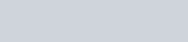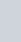A number is selected at random from the numbers 3, 5, 5, 7, 7, 7, 9, 9, 9, 9
Question:

A number is selected at random from the numbers 3, 5, 5, 7, 7, 7, 9, 9, 9, 9 The probability that the selected number is their average is

(a) $\frac{1}{10}$

(b) $\frac{3}{10}$

(c) $\frac{7}{10}$

(d) $\frac{9}{10}$

Solution:

GIVEN: A number is selected from the numbers 3,5,5,7,7,7,9,9,9,9

TO FIND: Probability that the selected number is the average of the numbers

Total numbers are 10

Average of numbers

$=\frac{3+5+5+7+7+7+9+9+9+9}{10}$

$=\frac{70}{10}$

$=7$

Total numbers of numbers which are average of these numbers are 3

We know that PROBABILITY =Hence Probability that the selected number is the average of the numbers isHence the correct option is